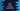# Java program to check if a string is palindrome or not## Java program to check if a string is palindrome or not:

In this post, we will learn how to check if a string is palindrome or not in Java. A string is called palindrome if it is equal to its reverse or if we reverse the characters of a string, it will be equal to the original string.

For example, reviver, racecar, madam, refer, level, etc. are palindrome strings.

This program will work for both uppercase and lowercase characters. For example, RaceCar, RACECAR both are palindrome strings. So, we will have to convert the string to uppercase or lowercase before we check for palindrome.

### Algorithm 1: By using two pointers:

With this approach, we will use two pointer variables. In the beginning, one will point to the start of the string and another will point to the end of the string. The start pointer will keep on incrementing on each step and the end pointer will keep on decrementing.

In each step, we will compare the characters pointed by these two pointers. If at any point, the character pointed by the left pointer is not equal to the character pointed by the right pointer, it will print that it is not a palindrome. Otherwise, if the left pointer value is greater than the right pointer value at some point,

Following are the steps:

• Initialize two variables. One as 0 and another as string length - 1
• Run a loop. It will run until the first variable is smaller than the second variable.
• Inside the loop, check the characters pointed by these two variables.
• If the characters are not equal, it is not a palindrome string. Return false.
• Increment the left variable by 1 and decrement the right variable by 1 at the end of each loop iteration.
• If the loop ends, i.e. no characters are found which are not equal, return true.

### Java program with two pointers approach:

Let’s write down the Java program that uses the above approach:

``````class Main {
public static boolean isPalindrome(String givenString) {
String str = givenString.toLowerCase();
int start = 0, end = str.length() - 1;

while (start < end) {
if (str.charAt(start) != str.charAt(end)) {
return false;
}
start++;
end--;
}
return true;
}

public static void main(String[] args) {
String[] arr = {"RaceCar", "hello", "reViver", "madAm", "man", "mam", "REFER", "level", "abcdedcba"};

for (String s : arr) {
System.out.println(s + " : " + isPalindrome(s));
}
}
}``````

Here,

• isPalindrome method is used to check if a string is palindrome or not. This method takes a string as the parameter and returns one boolean value. It returns true if it is palindrome, else it returns false.
• We are converting the string to lowercase in the beginning. This value is stored in str.
• The start variable is initialized as 0 and end variable is initialized as string length - 1.
• The while loop will run until the value of start is smaller than end. Inside this loop, we are comparing the characters at position start and end. If both are not equal, it returns false.
• At the end of each iteration, we are incrementing the value of start by 1 and decrementing the value of end by 1.
• Once the while loop ends, we are returning true.
• This program is checking for all the strings in arr.

If you run this program, it will print:

``````RaceCar : true
hello : false
reViver : true
man : false
mam : true
REFER : true
level : true
abcdedcba : true``````

### Method 2: By reversing the string:

We can also reverse the string and compare it with the original string. To reverse a string in Java, we can convert the string to a StringBuilder object and reverse it by using the reverse() method. StringBuilder objects can be converted to a string object by using toString() method.

Let’s write down the program to check how it works:

``````class Main {
public static boolean isPalindrome(String givenString) {
String str = givenString.toLowerCase();
return str.equals(new StringBuilder(str).reverse().toString());
}

public static void main(String[] args) {
String[] arr = {"RaceCar", "hello", "reViver", "madAm", "man", "mam", "REFER", "level", "abcdedcba"};

for (String s : arr) {
System.out.println(s + " : " + isPalindrome(s));
}
}
}``````

Here, we are comparing str with new StringBuilder(str).reverse().toString(), which is the reverse of the string str.

It will give a similar output.